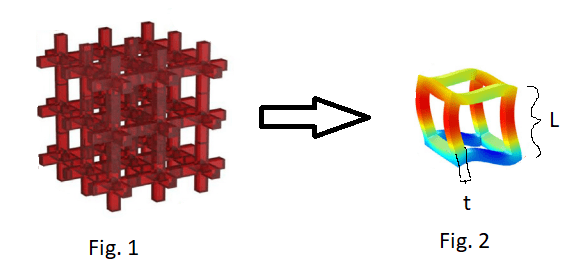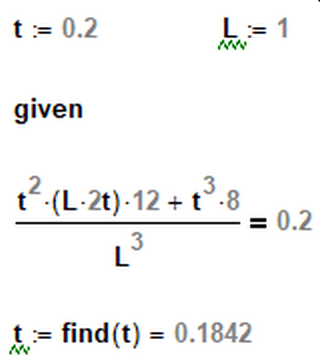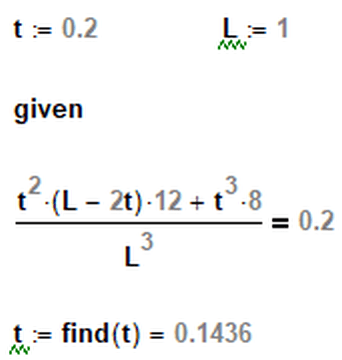×
INTELLIGENT WORK FORUMS
FOR ENGINEERING PROFESSIONALS

Are you an
Engineering professional?
Join Eng-Tips Forums!
• Talk With Other Members
• Be Notified Of Responses
• Keyword Search
Favorite Forums
• Automated Signatures
• Best Of All, It's Free!

*Eng-Tips's functionality depends on members receiving e-mail. By joining you are opting in to receive e-mail.

#### Posting Guidelines

Promoting, selling, recruiting, coursework and thesis posting is forbidden.

# Is this the right way to calculate volume fraction?

## Is this the right way to calculate volume fraction?

(OP)
I exploit the symmetry of fig.1 to simplify it to fig.2. This figure has a volume fraction of 0.2, and I need to find a relation between t and L. Have I done it right?

Volume of cube in the middle: (L-2*t)^3

And 4 rectangular plates around the cube in the middle: t*(L-2*t)^2

Volume of fig 2 is then: L^3 – ((L-2*t)^3 + t*(L-2*t)^2)

So volume fraction is that volume divided by the unit cell cube volume, giving:

(L^3 – ((L-2*t)^3 + t*(L-2*t)^2))/L^3 = 0.2

Which gives the relation:

t = 0.0428787 L### RE: Is this the right way to calculate volume fraction?

Figure 2 is not a unit cell, in that you cannot stack those next to each other to produce figure 1.

The corners of the unit cell are in the center of where the rods intersect.

Also, your last equation cannot be close to correct because the relationship between t and L is not a constant. L and t can be any two numbers, as long as L>2t.

### RE: Is this the right way to calculate volume fraction?

He's given a fixed volume fraction, though. Nevertheless, the final equation is wrong for another reason, namely, the numerator should only contain the volume associated with the beams. Something like below. There are 12 beams and 8 corner cubesTTFN (ta ta for now)
I can do absolutely anything. I'm an expert! https://www.youtube.com/watch?v=BKorP55Aqvg
FAQ731-376: Eng-Tips.com Forum Policies forum1529: Translation Assistance for Engineers Entire Forum list http://www.eng-tips.com/forumlist.cfm

### RE: Is this the right way to calculate volume fraction?

"(L.2t)" should be (L-2t)

L is CL to CL and t is the width of an arm, and the arms are in the edges of the cube, then the empty central cube is (L-t)^3 ... t = 2*(t/2)
and there are 6 side (face) voids (L-t)^2*(t/2) ...
(L-t)^3+6*((L-t)^2*(t/2)) = 0.8*L^3
(L-t)^2*((L-t)+3t) = 0.8*L^3 or
(1-(t/L))^2*(1+2*(t/L)) = 0.8
t/L = 0.287141
(if an arm is 2t wide, then t = 0.14357

or the filled space is 20% ...
4*L*(t/2)^2 + 8*(L-t)*(t/2)^2 = 0.2*L^3
(t/L)^2 *(1+2*(1-(t/L))) = 0.2

if the arms are central to the cube (but then there's on "central void") ...
3*L*t^2 - 2*t^3 = 0.2*L^3
3(t/L)^2 -2(t/L)^3 = 0.2
huh!, exactly the same ...

### RE: Is this the right way to calculate volume fraction?

Correction to above; thank rb1957TTFN (ta ta for now)
I can do absolutely anything. I'm an expert! https://www.youtube.com/watch?v=BKorP55Aqvg
FAQ731-376: Eng-Tips.com Forum Policies forum1529: Translation Assistance for Engineers Entire Forum list http://www.eng-tips.com/forumlist.cfm

### RE: Is this the right way to calculate volume fraction?

so you're defining the arms as 2t wide ?
OP's sketch defines as "t" wide.

### RE: Is this the right way to calculate volume fraction?

ok, but you can see the confusion.

for my money the interesting thing was modelling as a central stiffener gave the same result and a simple neat expression ...
3(t/L)^2 -2(t/L)^3 = 0.2

### RE: Is this the right way to calculate volume fraction?

Actually, the OP's image shows his "unit cell," wherein I presumed that the "t" was one-half of the thickness of the beams in the full-up structure, so I was simply following his lead.

TTFN (ta ta for now)
I can do absolutely anything. I'm an expert! https://www.youtube.com/watch?v=BKorP55Aqvg
FAQ731-376: Eng-Tips.com Forum Policies forum1529: Translation Assistance for Engineers Entire Forum list http://www.eng-tips.com/forumlist.cfm

### RE: Is this the right way to calculate volume fraction?

yeah, but he shows the width of a leg being "t".

### RE: Is this the right way to calculate volume fraction?

to the OP ...

"Volume of cube in the middle: (L-2*t)^3" ... no, if L is CL to CL then it's L-2(*(t/2) = (L-t)^3

"And 4" ... no, there are 6 (one at each face of the cube) ...
"rectangular plates around the cube in the middle: t*(L-2*t)^2" ... no, t/2*(L-t)^2

### RE: Is this the right way to calculate volume fraction?

The unit cell would have the legs "t" being half the width of the full structure's, I think, since one would slice the structure right down middle of the width of each full sized leg

TTFN (ta ta for now)
I can do absolutely anything. I'm an expert! https://www.youtube.com/watch?v=BKorP55Aqvg
FAQ731-376: Eng-Tips.com Forum Policies forum1529: Translation Assistance for Engineers Entire Forum list http://www.eng-tips.com/forumlist.cfm

#### Red Flag This Post

Please let us know here why this post is inappropriate. Reasons such as off-topic, duplicates, flames, illegal, vulgar, or students posting their homework.

#### Red Flag Submitted

Thank you for helping keep Eng-Tips Forums free from inappropriate posts.
The Eng-Tips staff will check this out and take appropriate action.

#### Resources

Low-Volume Rapid Injection Molding With 3D Printed Molds
Learn methods and guidelines for using stereolithography (SLA) 3D printed molds in the injection molding process to lower costs and lead time. Discover how this hybrid manufacturing process enables on-demand mold fabrication to quickly produce small batches of thermoplastic parts. Download Now
Examine how the principles of DfAM upend many of the long-standing rules around manufacturability - allowing engineers and designers to place a partâ€™s function at the center of their design considerations. Download Now
Industry Perspective: Education and Metal 3D Printing
Metal 3D printing has rapidly emerged as a key technology in modern design and manufacturing, so itâ€™s critical educational institutions include it in their curricula to avoid leaving students at a disadvantage as they enter the workforce. Download Now

Close Box

# Join Eng-Tips® Today!

Join your peers on the Internet's largest technical engineering professional community.
It's easy to join and it's free.

Here's Why Members Love Eng-Tips Forums:

•Talk To Other Members
• Notification Of Responses To Questions
• Favorite Forums One Click Access
• Keyword Search Of All Posts, And More...

Register now while it's still free!